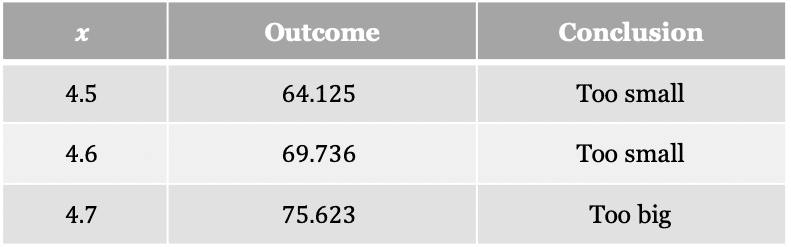# Solving Cubic Equations

GCSELevel 6-7WJEC

## Solving Cubic Equations

Solving higher order equations such as cubics can pose many challenges, the normal way of dealing with these is via trial and improvement.

Level 6-7GCSEWJEC

## Trial and Improvement Process

Trial and improvement is the process of taking an educated guess, seeing what result you get, and then improving on your previous guess with a new one.

Example: The solution to the equation $x^3-6x=72$ lies between $4$ and $5$. Use trial and improvement to find a solution correct to one decimal place.

Step 1: Make an educated guess, here choose $x=4.5$ since this is the middle of our range. This the first “trial”.

Step 2:  Substitute the trial into the equation

$(4.5)^3-6(4.5)=64.125$

Step 3: Improve on the previous trial, here choose $x=4.6$ since the last result was too small.

$(4.6)^3-6(4.6)=69.736$

This result was too small.

Step 4: Repeat step 3 until you get the opposite outcome, in this case look for a result that is too big. The table shows the various trials and conclusions from each trial.Step 5: Decide which solution to use. Since $4.6$ was too small and $4.7$ was too big, we must test $4.65$ as this is halfway in between.

$(4.65)^3-6(4.65)=72.644625$

Since this is too big, we must choose $x=4.6$ as the solution.

Level 6-7GCSEWJEC

## Solving Cubic Equations Example Questions

Start at $0.5$ since this is the middle of the range.

Create a table of results

 $x$ Outcome Conclusion $0.5$ $3.75$ Too small $0.6$ $4.632$ Too small $0.7$ $5.586$ Too small $0.8$ $6.624$ Too small $0.9$ $7.758$ Too big

Since $0.8$ is too small and $0.9$ is too big, we must trial $0.85$

$2(0.85)^3+7(0.85)=7.17825$

Since this is too big, we choose $x=0.8$ as the solution.

Start at $-3.5$ since this is the middle of the range.

Create a table of results

 $x$ Outcome Conclusion $-3.5$ $10.625$ Too big $-3.6$ $7.944$ Too big $-3.7$ $5.047$ Too big $-3.8$ $1.928$ Too big $-3.9$ $-1.419$ Too small

Since $-3.9$ is too small and $-3.8$ is too big, we must trial $-3.85$

$(-3.85)^3+11(-3.85)+15=0.283375$

Since this is too big, we choose $x=-3.9$ as the solution.

Start at $1.5$ since this is the middle of the range.

Create a table of results

 $x$ Outcome Conclusion $1.5$ $-1.125$ Too small $1.6$ $0.048$ Too big

Since $1.5$ is too small and $1.6$ is too big, we must trial $1.55$

$3(1.55)^3+(1.55)^2-12(1.55)+6=-0.575875$

Since this is too small, we choose $x=1.6$ as the solution.

## You May Also Like...### MME Learning Portal

Online exams, practice questions and revision videos for every GCSE level 9-1 topic! No fees, no trial period, just totally free access to the UK’s best GCSE maths revision platform.

£0.00